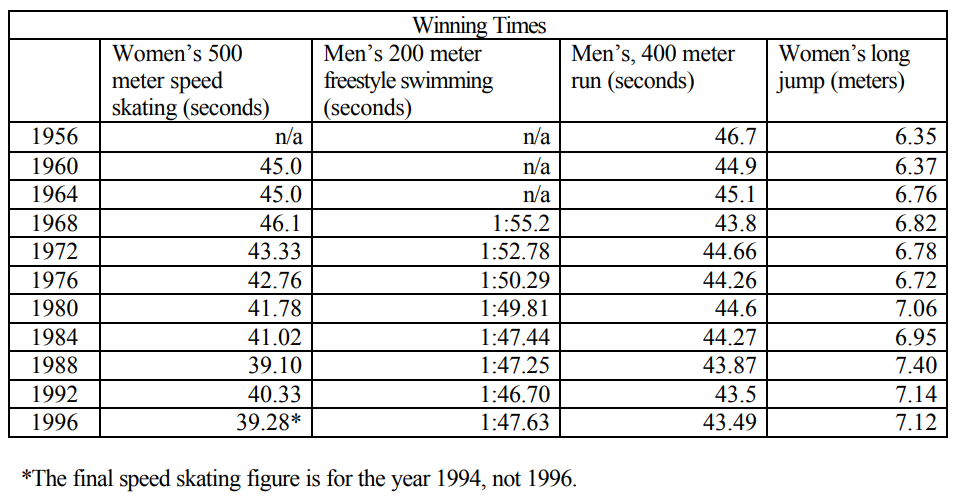Subject:
Mathematics

Topics: AlgebraPatternsFunctions and RelationsLinear Equations and FunctionsQuadratic Relations and FunctionsExponential Equations and Functions
Common Core State Standard: 6.EE.9, 8.F.1, N-Q.1, A-CED.2, A-REI.10, A-REI.11, F-IF.1, F-IF.2, F-IF.4, F-IF.5, F-IF.7a,
Concepts:

· Function

Knowledge and Skills:

· Can extrapolate a graph when a pattern exists
· Can plot a point in a two-dimensional coordinate system, given the coordinates, or determine the coordinates of a given point
· Can determine the equation of a linear function that closely matches a set of points
· Can determine a quadratic function that closely matches a set of points
· Can determine an exponential function that closely matches a set of points

Lesson:

Procedure: This project should be done by students individually or in teams of two..

Distribute the handout and discuss it. Ensure that students understand the assignment..

You may wish to leave it to the students to work out a technique for predict future values, or work out the procedure in class discussion and have the students apply it.

For less advanced students, a good technique is to simply graph the given data (making sure the horizontal axis extends from 1956 to around 2050) and then extend the curve visually.

More advanced students should be instructed to come up with a mathematical model* that most closely describes the given data, and use that to predict the value 40 years into the future.

*That is, a function chosen from among the ones the students have experience with: linear, quadratic, exponential, logarithmic, polynomial, or sinusoidal.

### Sports Today and Tomorrow

E-mail printout 10:48 am

To: Research team
From: Features editor
Subject: 40 years from now

A lot of magazines have a feature like “40 years ago” where you can find out about things that happened in the past. But I want to do something a little different: “40 years from now.”

Please look at the data below, which gives Olympic winning times for various sports for the last 40 years or so, and work out what you think the times will be 40 years from now.

You can pick two out of the four sports.

I don’t just want your opinion on this-—I need something that is mathematically accurate and I need an explanation of how you got your numbers so we can explain it to our readers. Your explanation should include diagrams or graphs if possible—readers like pictures.

By the way, I expect you will see some interesting patterns in these numbers. Let me know what they are because we may want to do a separate story on that.

Janet